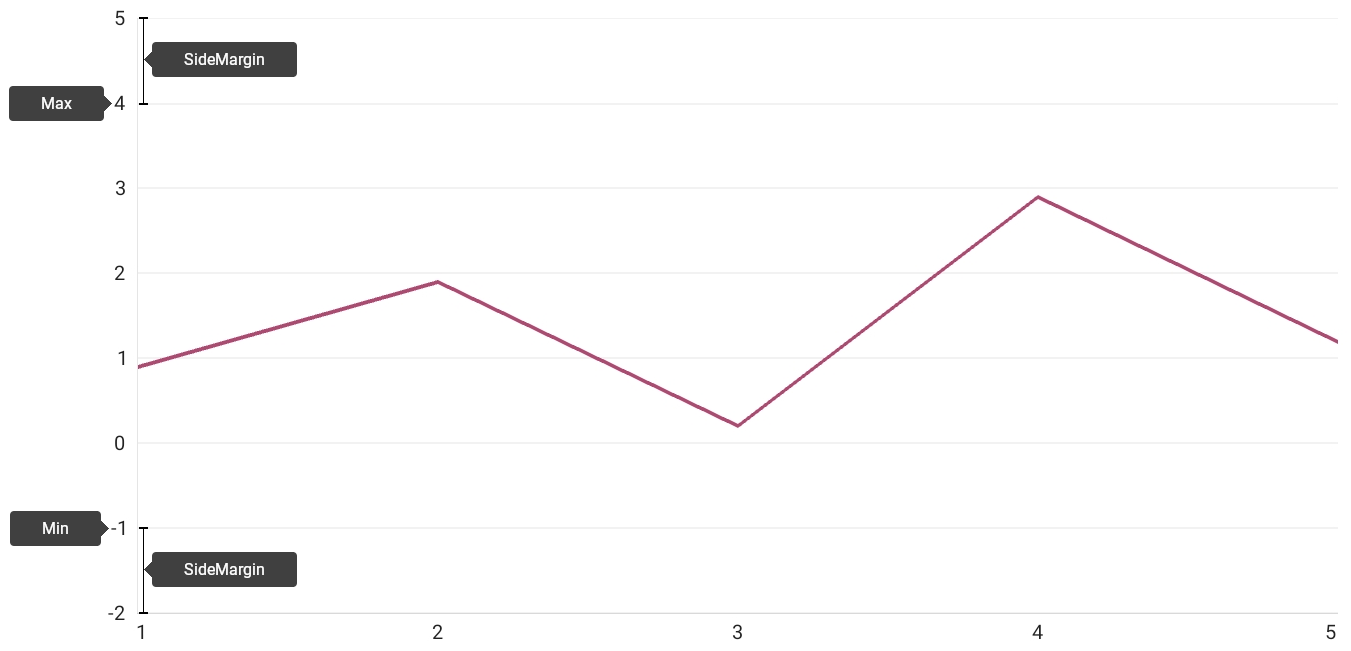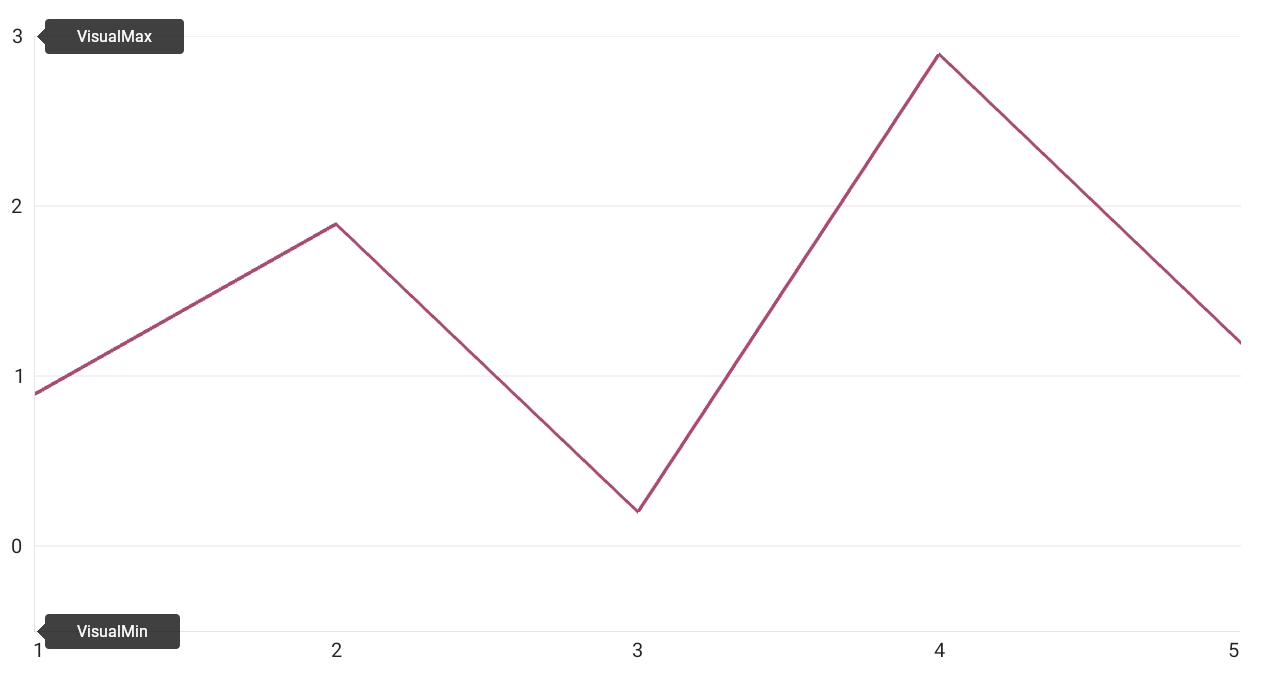# NumericRange Class

Stores range settings of NumericAxisX and NumericAxisY.

Namespace: DevExpress.Maui.Charts

Assembly: DevExpress.Maui.Charts.dll

NuGet Package: DevExpress.Maui.Charts

## Declaration

``````public class NumericRange :
RangeBase``````

The following members return NumericRange objects:

## Example

This example shows how to configure the numeric y-axis scale range. To do this, assign a `NumericRange` object with the specified properties to the NumericAxisY.Range property.

Whole Range and Side Margins

1. Use the Min and Max properties to specify the whole range of the axis scale. A chart does not visualize data that is out of this range. An end user can scroll the chart through this range when scrolling is enabled for the y-axis (ChartView.AxisYNavigationMode).

2. Use the RangeBase.SideMargin property to specify the value of indents on both sides of the axis.

``````<dxc:ChartView>
<dxc:ChartView.AxisY>
<dxc:NumericAxisY>
<dxc:NumericAxisY.Range>
<dxc:NumericRange Min="-1" Max="4" SideMargin="1"/>
</dxc:NumericAxisY.Range>
</dxc:NumericAxisY>
</dxc:ChartView.AxisY>
</dxc:ChartView>
``````Visual Range

Use the VisualMin and VisualMax properties to set an axis scale range currently displayed on a screen.

``````<dxc:ChartView>
<dxc:ChartView.AxisY>
<dxc:NumericAxisY>
<dxc:NumericAxisY.Range>
<dxc:NumericRange VisualMin="-0.5" VisualMax="3"/>
</dxc:NumericAxisY.Range>
</dxc:NumericAxisY>
</dxc:ChartView.AxisY>
</dxc:ChartView>
``````